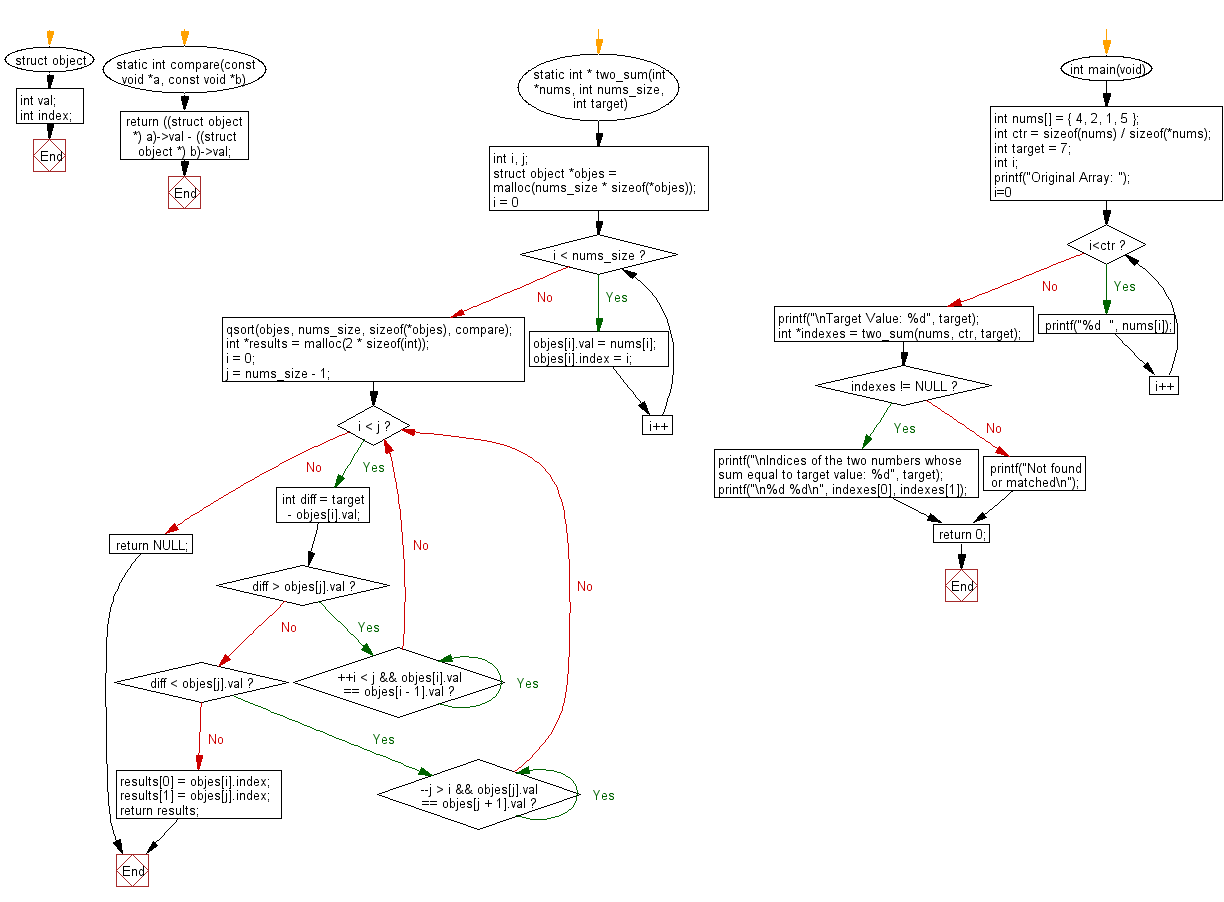﻿ C Program: Total of two indices values equal a target# C Exercises: Get the indices of the two numbers of a given array of integers

## C Programming Practice: Exercise-1 with Solution

Write a C programming to get the indices of the two numbers of a given array of integers, such that the sum of the two numbers equal to a specific target.

C Code:

``````#include <stdio.h>
#include <stdlib.h>

struct object {
int val;
int index;
};

static int compare(const void *a, const void *b)
{
return ((struct object *) a)->val - ((struct object *) b)->val;
}

static int * two_sum(int *nums, int nums_size, int target)
{
int i, j;
struct object *objes = malloc(nums_size * sizeof(*objes));
for (i = 0; i < nums_size; i++) {
objes[i].val = nums[i];
objes[i].index = i;
}
qsort(objes, nums_size, sizeof(*objes), compare);

int *results = malloc(2 * sizeof(int));
i = 0;
j = nums_size - 1;
while (i < j) {
int diff = target - objes[i].val;
if (diff > objes[j].val) {
while (++i < j && objes[i].val == objes[i - 1].val) {}
} else if (diff < objes[j].val) {
while (--j > i && objes[j].val == objes[j + 1].val) {}
} else {
results = objes[i].index;
results = objes[j].index;
return results;
}
}
return NULL;
}

int main(void)
{
int nums[] = { 4, 2, 1, 5 };
int ctr = sizeof(nums) / sizeof(*nums);
int target = 7;
int i;
printf("Original Array: ");
for(i=0; i<ctr; i++)
{
printf("%d  ", nums[i]);
}
printf("\nTarget Value: %d", target);
int *indexes = two_sum(nums, ctr, target);
if (indexes != NULL) {
printf("\nIndices of the two numbers whose sum equal to target value: %d", target);
printf("\n%d %d\n", indexes, indexes);
}
else
{
}

return 0;
}

``````

Sample Output:

```Original Array: 4  2  1  5
Target Value: 7
Indices of the two numbers whose sum equal to target value: 7
1 3
```

Pictorial Presentation:Flowchart:## C Programming Code Editor:

What is the difficulty level of this exercise?

Test your Programming skills with w3resource's quiz.

﻿

## C Programming: Tips of the Day

Maximum value of int:

In C:

```#include <limits.h>
then use
int imin = INT_MIN; // minimum value
int imax = INT_MAX;```

or

```#include <float.h>

float fmin = FLT_MIN;  // minimum positive value
double dmin = DBL_MIN; // minimum positive value

float fmax = FLT_MAX;
double dmax = DBL_MAX;```

Ref : https://bit.ly/3fi8yk9